Ex 15.1

Chapter 15 Class 10 Probability
Serial order wise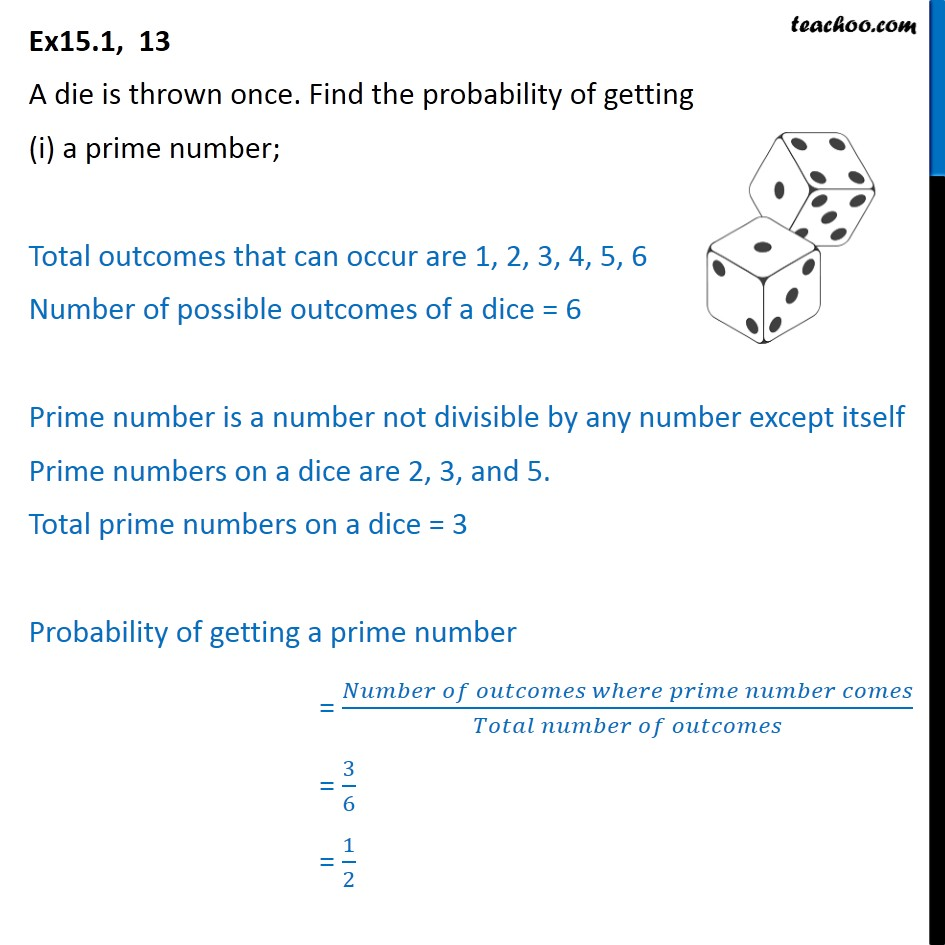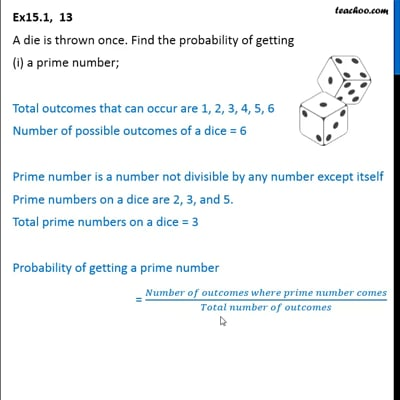This video is only available for Teachoo black users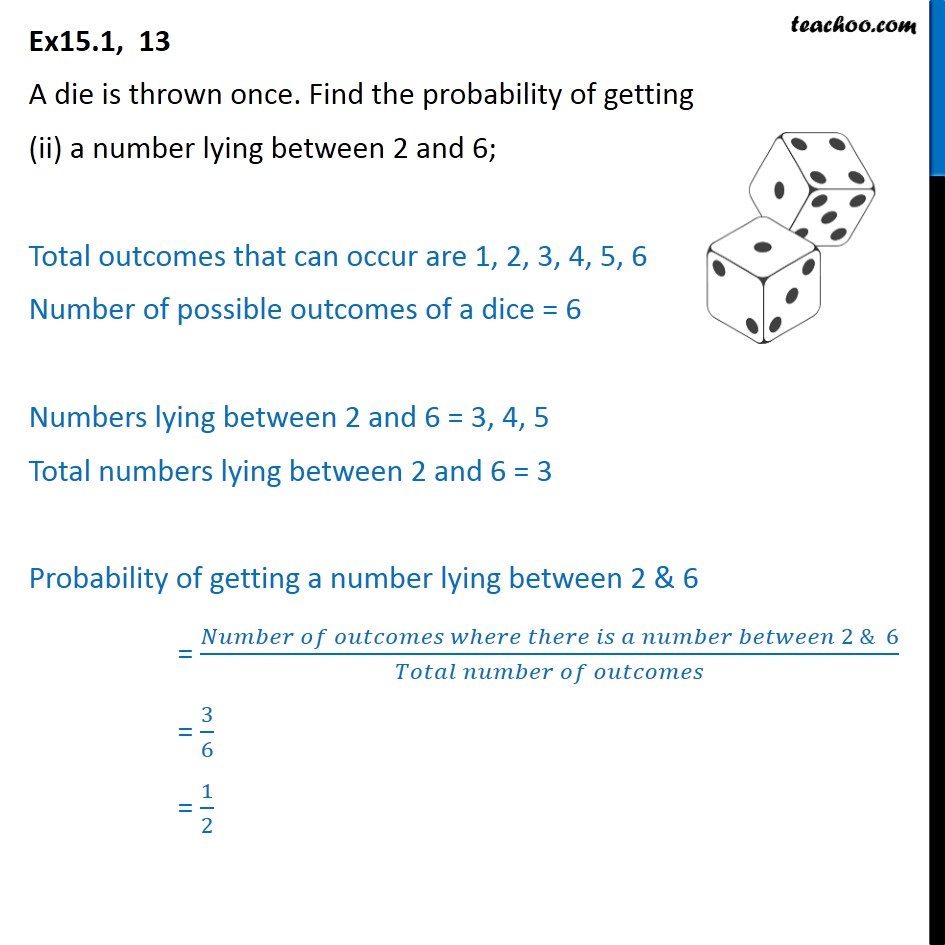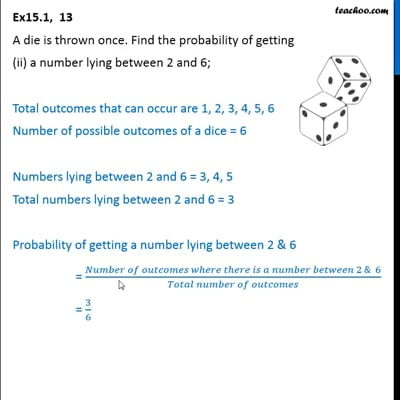This video is only available for Teachoo black users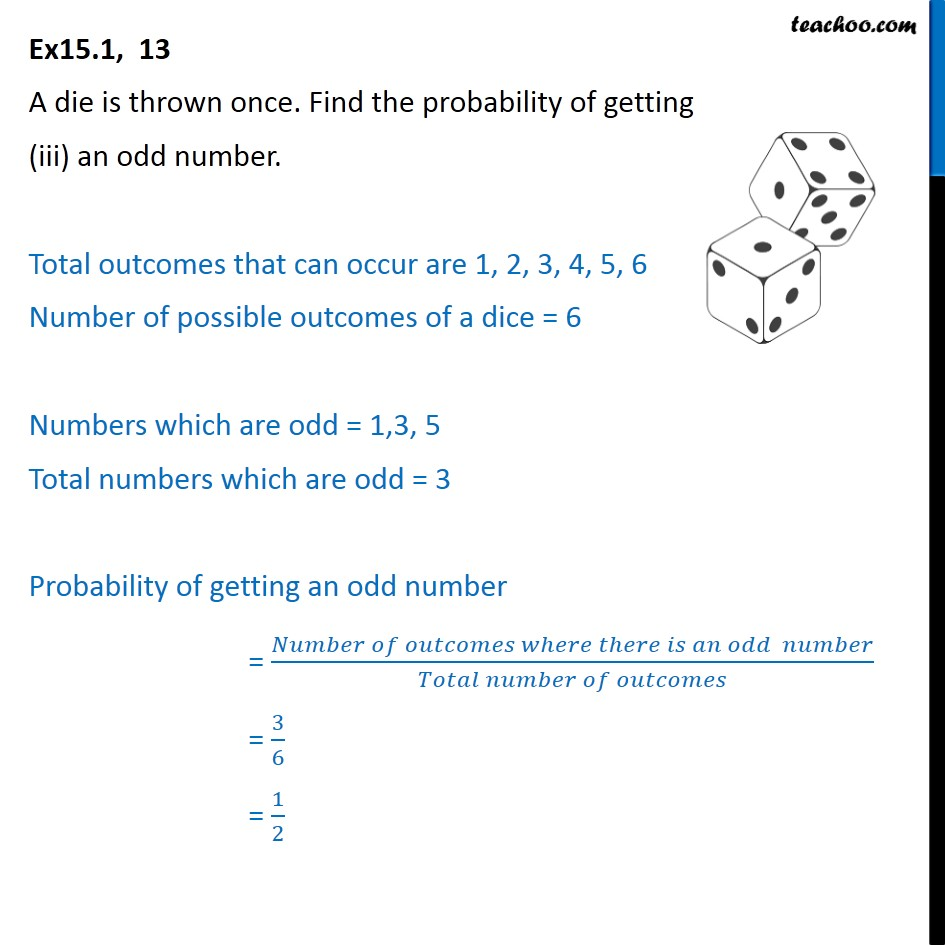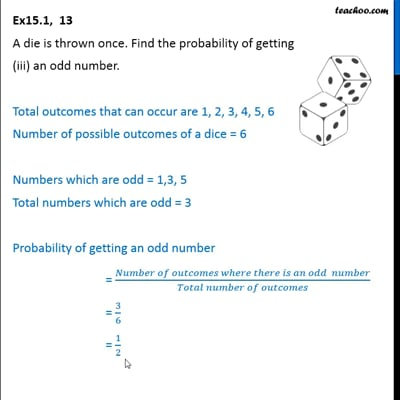This video is only available for Teachoo black users

Introducing your new favourite teacher - Teachoo Black, at only ₹83 per month

### Transcript

Ex15.1, 13 A die is thrown once. Find the probability of getting (i) a prime number; Total outcomes that can occur are 1, 2, 3, 4, 5, 6 Number of possible outcomes of a dice = 6 Prime number is a number not divisible by any number except itself Prime numbers on a dice are 2, 3, and 5. Total prime numbers on a dice = 3 Probability of getting a prime number = (𝑁𝑢𝑚𝑏𝑒𝑟 𝑜𝑓 𝑜𝑢𝑡𝑐𝑜𝑚𝑒𝑠 𝑤ℎ𝑒𝑟𝑒 𝑝𝑟𝑖𝑚𝑒 𝑛𝑢𝑚𝑏𝑒𝑟 𝑐𝑜𝑚𝑒𝑠)/(𝑇𝑜𝑡𝑎𝑙 𝑛𝑢𝑚𝑏𝑒𝑟 𝑜𝑓 𝑜𝑢𝑡𝑐𝑜𝑚𝑒𝑠) = 3/6 = 1/2 Ex15.1, 13 A die is thrown once. Find the probability of getting (ii) a number lying between 2 and 6; Total outcomes that can occur are 1, 2, 3, 4, 5, 6 Number of possible outcomes of a dice = 6 Numbers lying between 2 and 6 = 3, 4, 5 Total numbers lying between 2 and 6 = 3 Probability of getting a number lying between 2 & 6 = (𝑁𝑢𝑚𝑏𝑒𝑟 𝑜𝑓 𝑜𝑢𝑡𝑐𝑜𝑚𝑒𝑠 𝑤ℎ𝑒𝑟𝑒 𝑡ℎ𝑒𝑟𝑒 𝑖𝑠 𝑎 𝑛𝑢𝑚𝑏𝑒𝑟 𝑏𝑒𝑡𝑤𝑒𝑒𝑛 2 & 6)/(𝑇𝑜𝑡𝑎𝑙 𝑛𝑢𝑚𝑏𝑒𝑟 𝑜𝑓 𝑜𝑢𝑡𝑐𝑜𝑚𝑒𝑠) = 3/6 = 1/2 Ex15.1, 13 A die is thrown once. Find the probability of getting (iii) an odd number. Total outcomes that can occur are 1, 2, 3, 4, 5, 6 Number of possible outcomes of a dice = 6 Numbers which are odd = 1,3, 5 Total numbers which are odd = 3 Probability of getting an odd number = (𝑁𝑢𝑚𝑏𝑒𝑟 𝑜𝑓 𝑜𝑢𝑡𝑐𝑜𝑚𝑒𝑠 𝑤ℎ𝑒𝑟𝑒 𝑡ℎ𝑒𝑟𝑒 𝑖𝑠 𝑎𝑛 𝑜𝑑𝑑 𝑛𝑢𝑚𝑏𝑒𝑟)/(𝑇𝑜𝑡𝑎𝑙 𝑛𝑢𝑚𝑏𝑒𝑟 𝑜𝑓 𝑜𝑢𝑡𝑐𝑜𝑚𝑒𝑠) = 3/6 = 1/2